# simts Vignettes

## Time Series Simulation

In this section, we briefly list, describe, and provide the syntax used to simulate time series data using the simts package. The following list includes some basic models available in this package:

• White Noise WN()
• Quantization Noise QN()
• Random Walk RW()
• Drift DR()
• First-order Autoregressive AR1()
• Autoregressive AR()
• First-order Moving Average MA1()
• Moving Average Process MA()
• Gauss-Markov GM()
• Autoregressive Moving Average ARMA()
• Integrated Autoregressive Moving Average ARIMA()
• Seasonal Autoregressive Integrated Moving Average SARIMA()
• Seasonal Autoregressive Moving Average SARMA()
• Quantization Noise QN()

Quantization noise is a less known process that is used in engineering applications. It can be described in layperson terms as being a good estimator of a rounding error.

The code below shows how to call the function gen_gts(), which allows the user to generate samples from the above model specifications.

# Set seed for reproducibility
set.seed(1337)

# Number of observations
n = 10^4

# Generate a White Noise Process
wn = gen_gts(n, WN(sigma2 = 1))

# Generate a Quantization Noise
qn = gen_gts(n, QN(q2 = .5))

# Generate a Random Walk
rw = gen_gts(n, RW(gamma2 = .75)) 

By applying the plot() function on the result of a gen_gts() simulation, we can observe a visualization of our simulated data.

par(mfrow = c(3,1))
plot(wn)
plot(qn)
plot(rw)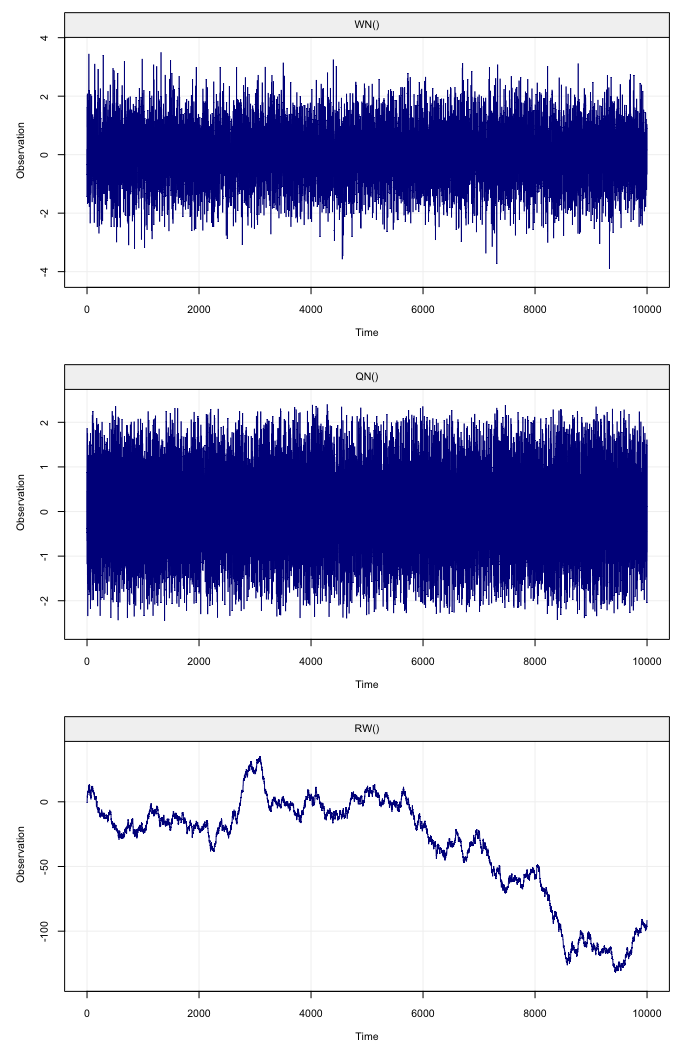Figure 1: Simulated white noise process (top panel), quantiation noise (middle panel) and random walk process (bottom panel)

Another example with a SARIMA model is given below:

# Generate an SARIMA(1,0,1)x(2,1,1)
sarima = gen_gts(n, SARIMA(ar = 0.3, i = 0, ma = -0.27,
sar = c(-0.12, -0.2), si = 1, sma = -0.9,
sigma2 = 1.5, s = 12))
# Plot simulation of SARIMA(1,0,1)x(2,1,1)
plot(sarima)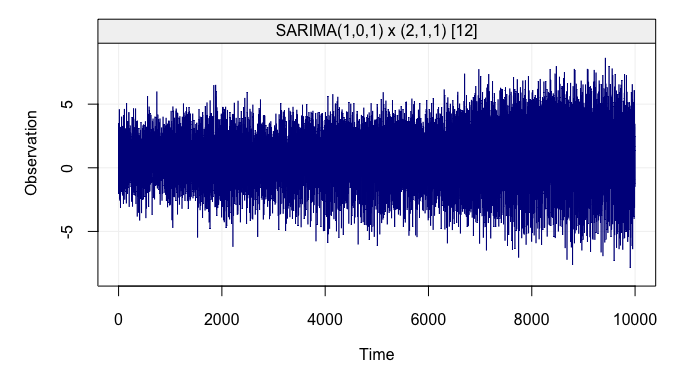Figure 2: Simulated SARIMA(1,0,1)x(2,1,1) process

The simts package therefore allows users to easily simulate from a wide variety of classical time series models, but does not limit itself to these models. Indeed, under some restrictions, these models can be combined in different ways to deliver many state-space (latent) models which can be represented as the sum of basic models.

simts’s user friendly interface allows for easy construction of such linear state-space models. In fact, to specify that a certain model is a combination of different models, all that is needed is the “+” symbol between them. For example, consider the following state-space model:

\begin{aligned} X_t &= X_{t-1} + \omega + U_t, \;\;\;\;\; U_t \sim \mathcal{N}(0,\gamma^2),\\ Y_t &= X_t + Z_t , \;\;\;\;\; Z_t \sim \mathcal{N}(0,\sigma^2), \end{aligned}

it is easy to see that this model is exactely equivalent to the sum of a random walk (with inivation variance $$\gamma^2$$), a linear drift (with slope $$\omega$$) and a white noise process (with variance $$\sigma^2$$). Therefore, it can easily be simulated as follows:

set.seed(1)
model = RW(gamma2 = 0.01) + DR(omega = 0.001) + WN(sigma2 = 1)
Yt = gen_gts(model, n = 10^3)
plot(Yt)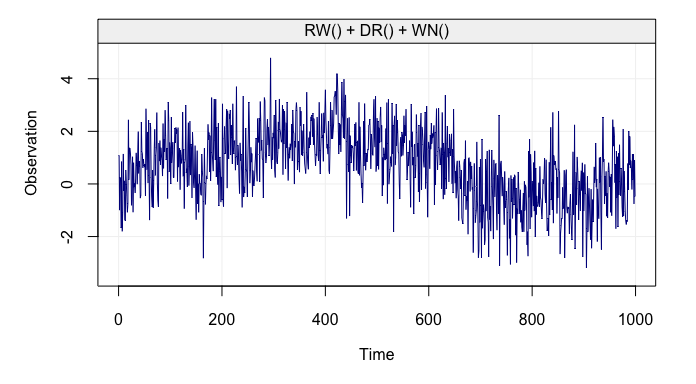Figure 3: Simulated state-space model (RW + WN + DR)

It is also possible to retrieve and visualize the three latent used to construct such state-space model using the function gen_lts() instead of gen_gts(), as follows:

set.seed(1)
model = RW(gamma2 = 0.01) + DR(omega = 0.001) + WN(sigma2 = 1)
Yt = gen_lts(model, n = 10^3)
plot(Yt)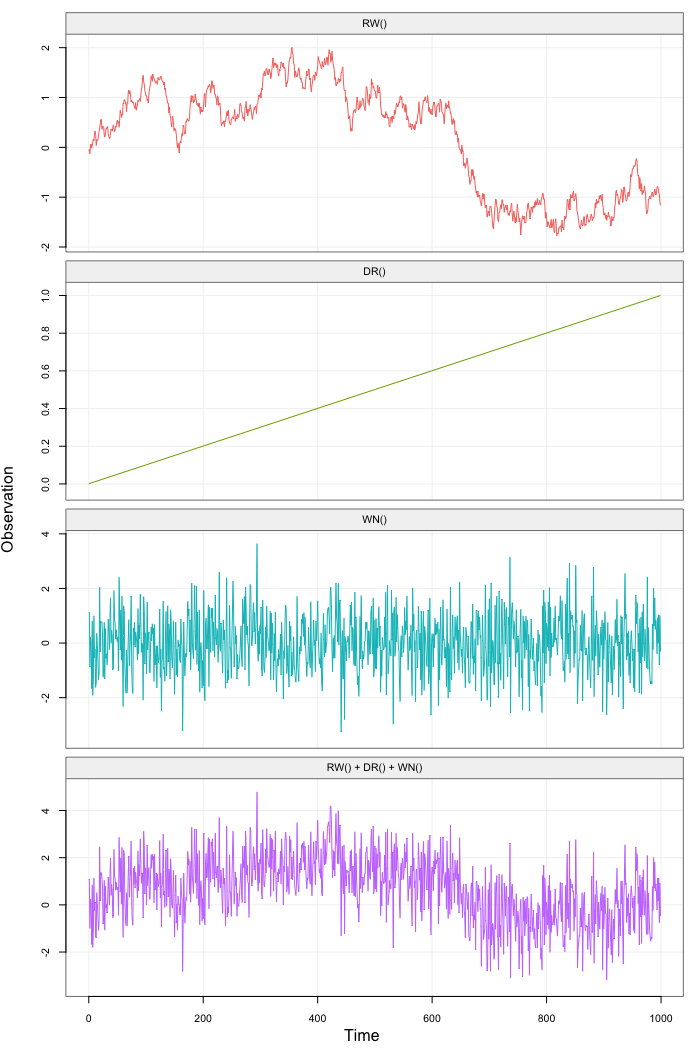Figure 4: Simulated state-space model (RW + WN + DR) showing latent processes

Consider another example, let us suppose that different AR(1) processes are present in a state-space model. The syntax to insert “k” of these models into the state-space model is k*AR1(). So, for example, the sum of three AR1 models, a random walk and a white noise process can be given by a simple expression: 3*AR1()+RW()+WN().

Examples of simulating such models are generated below.

# Generate a ARMA(2,1) + WN()
arma_wn_model =
ARMA(ar = c(0.9, -0.5), ma = 0.3, sigma2 = 1) +
WN(sigma = 4)
arma_wn_sim = gen_gts(n = n, model  = arma_wn_model)

# Plot simulation of ARMA(2,1) + WN()
plot(arma_wn_sim)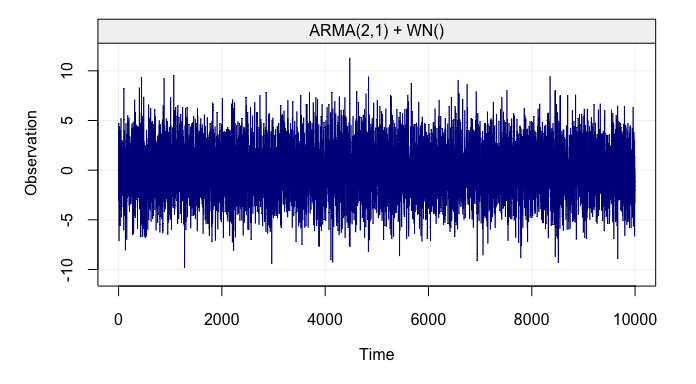Figure 5: Simulated ARMA(2,1) + WN() process

As mentioned earlier, simts provides a function specifically designed to generate and represent latent time series models: gen_lts(). This provides users the option to visualize a breakdown of the underlying processes by applying the plot() function on the result of gen_lts().

# Generate a SARMA() + WN()
sarma_wn_model =
SARMA(ar = 0, ma = 0, sar = 0.98, sma = 0, s = 10, sigma2 = 1) +
WN(sigma2 = 1)
sarma_wn_sim = gen_lts(n = 10^3, model = sarma_wn_model)

# Plot simulation of SARMA() + WN()
plot(sarma_wn_sim)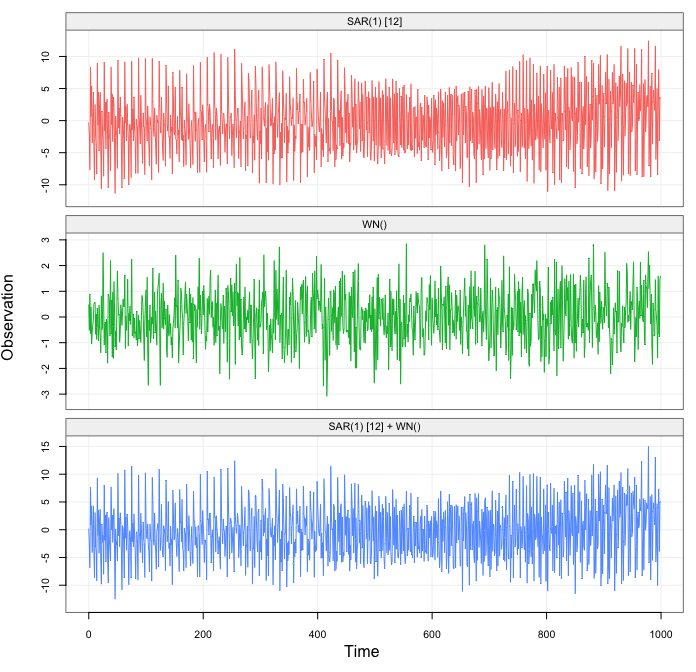Figure 6: Simulated SARMA(1,0) x (0,1) + WN(2) process with a breakdown of the underlying latent processes

To better visualize the contribution to each process by using the sam range on the “y-axis”. This can be done with the option fixed_range = TRUE as follows:

plot(sarma_wn_sim, fixed_range = TRUE)